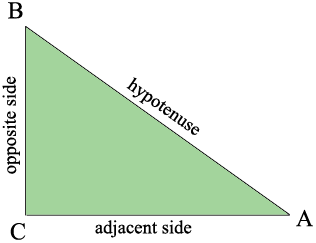SEARCH HOMEMath Central Quandaries & QueriesQuestion from Tizoc, a student: I am in a trig class and I have a conflict. When solving the length of a side, I know what trig function to use, but I do not know what angle to use in a calculator. To make this a little more understandable, if I have all the angles available in a right triangle and I use the tangent function, how do I know what to use? Here's what I do not know what to put in my calculator: Tan(?) Thanks in Advance!Hi Tizoc,

I'm not sure I understand your question but I'll give it a try. I expect that your textbook or teacher have told you that

$\tan(A) = \frac{\mbox{opposite}}{\mbox{adjacent}}.$

In my diagram of a right triangle, since I am interested in the angle at $A$ the side opposite to $A$ is $BC$ and the side adjacent to $A$ is $CA.$Suppose that measure of the angle at $A$ is $37^o .$ Suppose you also know that the length of $CA$ is $15$ centimeters then

$\tan(37^o) = \frac{\mbox{opposite}}{\mbox{adjacent}} = \frac{|BC|}{|CA|} = \frac{|BC|}{15},$

and hence

$|BC| = 15 \times \tan(37^o).$

Now it's time to use your calculator. Make sure it is set on degrees and input $\tan(37).$ My calculator gave me $\tan(37) = 0.7536$ and hence

$|BC| = 15 \times 0.7536 = 11.3 \mbox{ centimeters.}$

Write back if I haven't answered your question,
PennyMath Central is supported by the University of Regina and The Pacific Institute for the Mathematical Sciences.